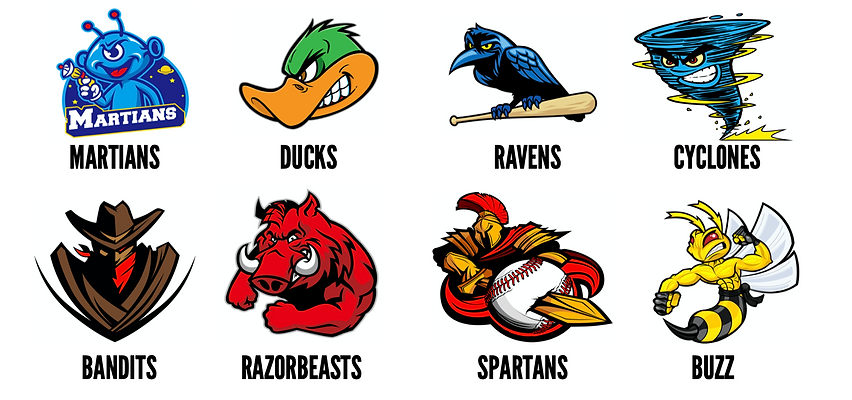The Math Baseball Project is a fun and exciting way to explore statistics.  One component of statistics is the understanding of probability.  Probability can be summed up in one word.  The word is chance.  Two components of probability are experimental probability and theoretical probability.  Both have major differences, obviously as implied in the name.  You will develop a strong conceptual understanding of the difference between the two probabilities while working collaboratively on this project.  Remember it is just a game!  It all comes down to chance.  Good luck and enjoy.While probability theory focuses on the likelihood of an event taking place, theoretical probability is all about the occurrence of an event based on all possible outcomes that are already known.  Theoretical probability uses the knowledge of the likely outcomes to find the different ways in which the event can occur.  It is the method of finding the probability of an event from a sample space of known and equally likely outcomes.

When all possible outcomes are equally likely, the theoretical probability of an event is the ratio of the number of favorable outcomes to the number of possible outcomes.